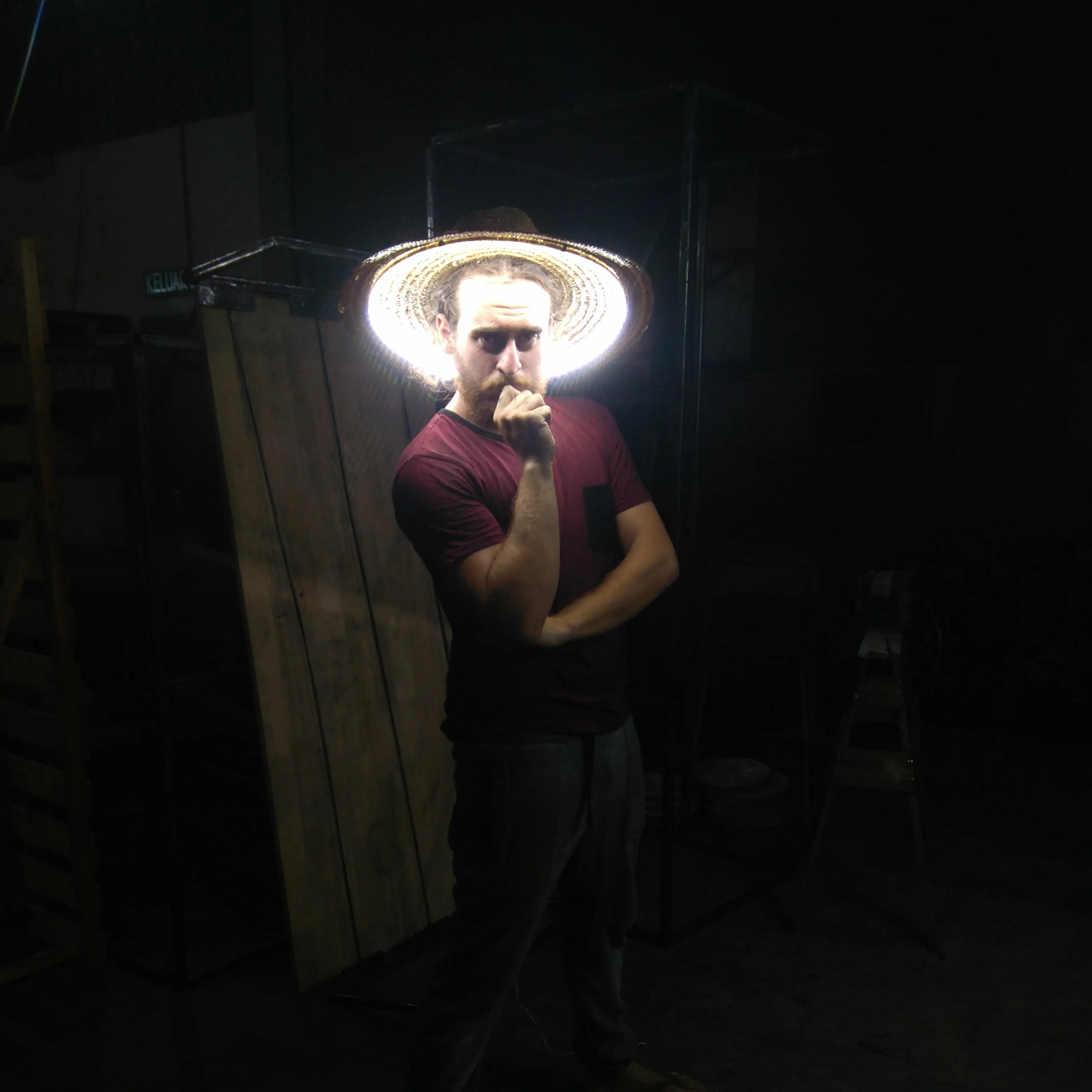# Ankle Air Guitar Pedal

Build an "invisibe" expression pedal for your guitar with MCP41010 and Arduino Nano. It's awesome and simple. May the funk be with you.

IntermediateWork in progress5 hours6,245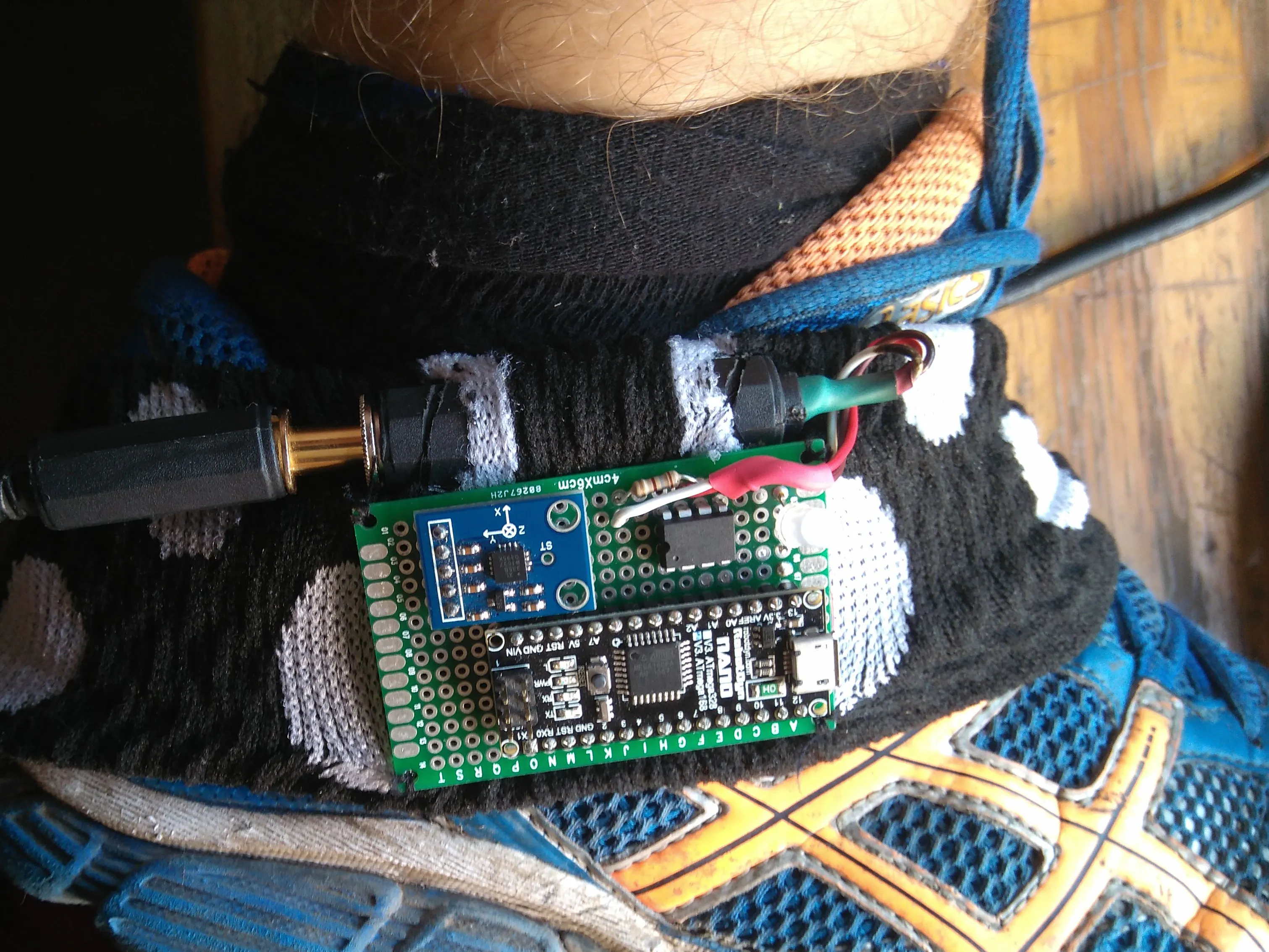## Things used in this project

### Hardware componentsArduino Nano R3 any arduino is okay, im thinking on using tinyduino
×1
 mpc41010 digital potenciometer
×1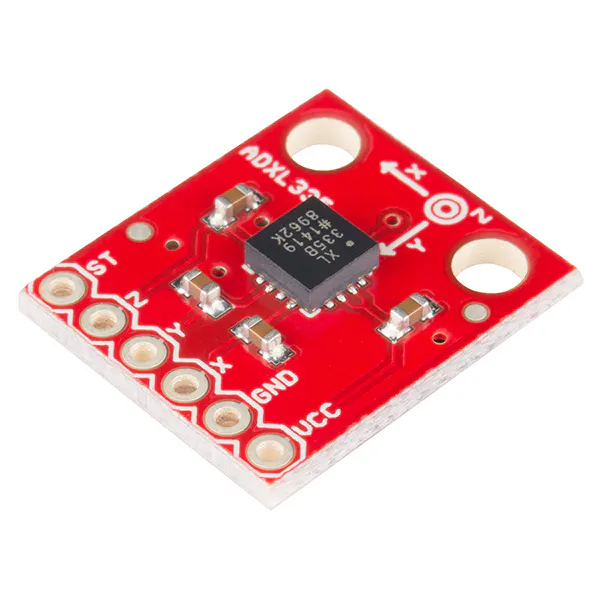SparkFun Triple Axis Accelerometer Breakout - ADXL335 any analogoutput accelerometer is okay
×1
 stereo audio jack 6,5 mm female adapter
×1
 stereo audio jack 6,5 mm cable
×1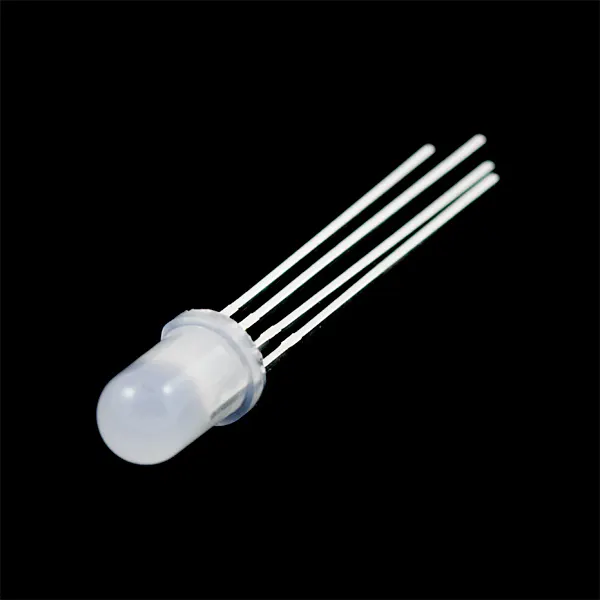RGB Diffused Common Cathode just for fun, no needed
×1

### Software apps and online servicesArduino IDE

## Schematics

### SCHEME ANKLE EXPRESSION PEDAL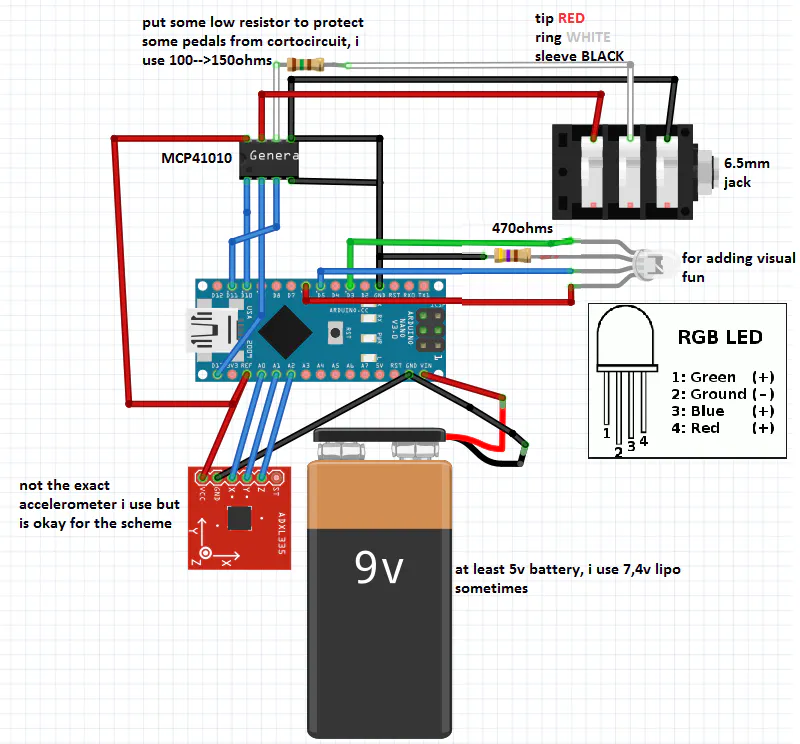## Code

### MAIN THING

Arduino
main raw program, the rgb led or any pwm signal is catastrophic fro the accelerometer readings (noise)
```#include <SPI.h>
#define debug //comment this line if you´re not gonna use the serial data with the arduino ide

#define CS 10 //chipselect

#define accelerometerX A0//analogpins for the accelerometer
#define accelerometerY A1
#define accelerometerZ A2

#define RED 6 //rgb pins
#define GREEN 3
#define BLUE 5

//MCP41010 chip´s connection
//        39ohms per step of the
//CS  10              U      vcc
//SCK 13 serial clock        PBO--UPRESISTOR
//SI  11 serial imput        PWO  INRESISTOR
//gnd                        PAO--BOTTOMRESISTOR

int datarray={0,0,0,0,0,0,1023,1023,1023,0,0,0,0,0,0};
int smoothingarray={0,0,0,0,0,0,0,0,0,0,0,0,0,0,0};
int rgb;
int i=0,chipvalue=0;
int ResistorValue=0;
void setup() {
//
//pinMode (GREEN, OUTPUT);//GREEN RGB (PWM)
//pinMode (BLUE, OUTPUT);//BLUE
//pinMode (RED, OUTPUT);//RED
//digitalWrite(GREEN, HIGH);//setting the led off at the begining
//digitalWrite (BLUE, HIGH);//
//digitalWrite (RED,  HIGH);//

pinMode (CS, OUTPUT);//chipselect for the  MCP41010 chip
pinMode (13, OUTPUT);//things are happening led
pinMode (accelerometerX, INPUT);//acelerometer´s inputs
pinMode (accelerometerY, INPUT);//
pinMode (accelerometerZ, INPUT);//
SPI.begin();
MCP41010Write(0);//init value horizontal foot

#ifdef debug
Serial.begin(9600);
#endif
}

void loop() {

comparemaxmin(datarray);

detectRange(datarray);

ResistorValue=map(datarray[datarray],datarray[datarray+6]+20,datarray[datarray+3]-10,0,255);//+20 to the min and -10 to the max for preventing unreachable edges

chipvalue =constrain(ResistorValue,0,255);

MCP41010Write(smoothing(chipvalue));

//  //map(value, fromLow, fromHigh, toLow, toHigh)
// for(i=0;i<=2;i++){
//  rgb[i]=map(datarray[i],datarray[i+6],datarray[i+3],0,255);
// }
////analogWrite(GREEN, rgb);//EL LED DA PROBLEMAS DE LECTURA ruido/////////////////////7
////analogWrite (BLUE, rgb);//
////analogWrite (RED,  rgb);//

#ifdef debug
Serial.print(datarray);
Serial.print(" ");
Serial.print(datarray);
Serial.print(" ");
Serial.print(datarray);

Serial.print("     /max ");
Serial.print(datarray);
Serial.print(" ");
Serial.print(datarray);
Serial.print(" ");
Serial.print(datarray);

Serial.print(" /min ");
Serial.print(datarray);
Serial.print(" ");
Serial.print(datarray);
Serial.print(" ");
Serial.print(datarray);

Serial.print("    //GAP :");
Serial.print(datarray);
Serial.print(" ");
Serial.print(datarray);
Serial.print(" ");
Serial.print(datarray);

//      Serial.print("/?? ");
//      Serial.print(datarray);
//      Serial.print(" ");
//      Serial.print(datarray);
//      Serial.print(" ");
//      Serial.print(datarray);

Serial.print("  //axis choose ");
Serial.print(datarray);

Serial.print("       //chip value ");
Serial.print(chipvalue);

Serial.println();
#endif
delay(100);//delay for stability
}

void comparemaxmin(int datarray[])
{
for(i=0;i<=2;i++)
datarray[i+3]=max(datarray[i],datarray[i+3]);

for(i=0;i<=2;i++)
datarray[i+6]=min(datarray[i],datarray[i+6]);

}

void detectRange(int datarray)
{
for(i=0;i<=2;i++)
{
datarray[i+9]=abs(abs(datarray[i+3])-abs(datarray[i+6]));
}
if(datarray>=datarray&&datarray>=datarray)//choosing the axis with the wider range
datarray=0;
if(datarray>=datarray&&datarray>=datarray)
datarray=1;
if(datarray>=datarray&&datarray>=datarray)
datarray=2;
}

void MCP41010Write(byte value) //speaking protocol with the mcp chip
{
// Note that the integer value passed to this subroutine
// is cast to a byte

digitalWrite(CS,LOW);
SPI.transfer(B00010001); // This tells the chip to set the pot
SPI.transfer(value);     // This tells it the pot position
digitalWrite(CS,HIGH);
}

int smoothing(int data){
int result,j;

for(j=0;j<=14;j++){
smoothingarray[j] =  smoothingarray[j+1];
}
smoothingarray= data;

result=(smoothingarray+smoothingarray+smoothingarray+smoothingarray+smoothingarray)/5;
constrain(result,0,255);
return result;
}
```

## Credits

### javier muñoz sáez

10 projects • 46 followers
Electronic engineer and sparky person. I make tutorials so my future self doesnt need to remember where he put the code